# Ages

John, Teresa, Daniel and Paul have summary 56 years. Their ages are in a ratio of 1:2:5:6. Determine how many years have each of them.

j =  4
t =  8
d =  20
p =  24

### Step-by-step explanation: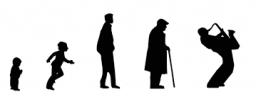Did you find an error or inaccuracy? Feel free to write us. Thank you!Tips to related online calculators
Check out our ratio calculator.

## Related math problems and questions:

• Moneys in triple ratioMilan, John and Lili have a total 344 euros. Their amounts are in the ratio 1:2:5. Determine how much each of them has?
• Jan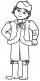Jan is 13 years and Peter 21 years old. For how many years will their ages in the ratio of 7:9?
• Ratio6 numbers are in the ratio 1:5:1:5:5:5. Their sum is 242. What are the numbers?
• Unknown number 5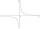Daniel thinks an integer. When he change this number at a ratio of 2:5, he got the number 2.8. Determine what number think Daniel.
• RatiosReduce the numbers: 50 in a 1:2 ratio 111 at a ratio of 2:3 70 at 10:50 560 at a ratio of 3:8
• PeppersIn the box are yellow (a), green (b), and red (c) peppers. Their amount is in a ratio of 2:4:1. Most are yellow peppers and green the least. Calculate the number of peppers each type if the total number of peppers is 70.
• Euros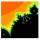Michal, Peter, John, and Lenka got together 2,400 euros. They share an amount in ratio 2:6:4:3. How many got each of them?
• Report 2A School reports students to teacher ratio of 6:1. If there are 45 teachers in the School, how many students are there?
• Election mathematics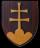In elections, 12 political parties received this shares of voters: party A 56.2 %party B 8.5 %party C 8.2 %party D 6.2 %party E 6.1 %party F 5.5 %party G 3.2 %party H 2.1 %party I 2 %party J 1 %party K 1 % Calculate what the shares acquired in the parliam
• String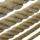Divide the string length 800 cm in ratio 1: 5
• Ratio three numbers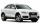Three numbers SUV are in the ratio 1:2:3. Their sum is 24. Find this numbers and write their add and sum.
• DonutsFind how many donuts each student will receive if you share 126 donuts in a ratio of 1:5:8
• Division of moneyCalculate how many euros have Matthew, Miriam, Lucy, Michael, Janka when together have 2,700 euros and the amounts are at a ratio of 1:5:6:7:8.
• Divide moneyDivide 1200 USD at a ratio of 1:2:3:4:5:6:9:10
• Line segmentCut a line segment of 15 cm into two line segments so that their lengths are in ratio 2:1. What length will each have?
• Five girls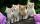Five girls are traveling by bus. Each holds in each hand two baskets. In each basket are four cats. Each cat has three kittens. Two girls exit the bus. How many legs are on the bus?
• Honza + Alice + Tonda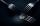Honza + Alice + Tonda have a total of 111 USD. The ratio between Honza and Alena is 5:6 and the ratio between Alice and Tonda is 4:5 How many money have each of them?